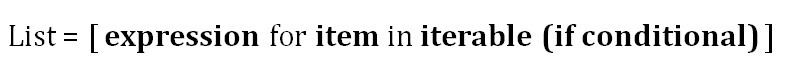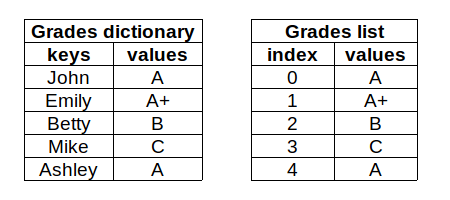# python必会的10个知识点

Python在数据科学生态系统中占据主导地位。我认为，占据主导地位的两大原因是相对容易学习和数据科学库的丰富选择。

Python是一种通用语言，因此它不仅仅用于数据科学，Web开发、移动应用程序和游戏开发也是Python的一些用例。

1.函数

``````def multiply(a, b):
return a * b

multiply(5, 4)
20``````

``````def is_long(word):
if len(word) > 8:
return f"{word} is a long word."

is_long("artificial")
'artificial is a long word.'``````

2.位置参数和关键字参数

• 位置参数仅由名称声明。

• 关键字参数由名称和默认值声明。

• 调用函数时，必须给出位置参数的值。否则，我们将得到一个错误。如果我们不为关键字参数指定值，它将采用默认值。

让我们用关键字参数重新定义multiply函数，这样我们就可以看到区别了。

``````def multiply(a=1, b=1):
return a * b

print(multiply(5, 4))
20

print(multiply())
1``````

3.args和*kwargs

函数是Python中的构建块。它们接受零个或多个参数并返回一个值。Python在参数如何传递给函数方面非常灵活。args和*kwargs使处理参数更容易、更清晰。

• *args允许函数接受任意数量的位置参数。

• 下面是一个简单的例子：

``````def addition(*args):
result = 0
for i in args:
result += i
return result

5

11``````
• **kwargs允许函数接受任意数量的关键字参数。

• 默认情况下，`**kwargs`是一个空字典。每个未定义的关键字参数都作为键值对存储在`**kwargs`字典中。

下面是一个简单的例子：

``````def arg_printer(a, b, option=True, **kwargs):
print(a, b)
print(option)
print(kwargs)

arg_printer(3, 4, param1=5, param2=6)
3 4
True
{'param1': 5, 'param2': 6}``````

https://towardsdatascience.com/10-examples-to-master-args-and-kwargs-in-python-6f1e8cc30749

4.类

面向对象编程（OOP）范式是围绕着拥有属于特定类型的对象的思想构建的。从某种意义上说，类型是解释我们的对象。

Python中的所有东西都是一种类型的对象，比如整数、列表、字典、函数等等。我们使用类定义一种对象类型。

类具有以下信息：

• 数据属性：创建类的实例需要什么

• 方法（即过程属性）：我们如何与类的实例交互。

• https://towardsdatascience.com/a-comprehensive-guide-for-classes-in-python-e6bb72a25a5e

5.列表

List是Python中的内置数据结构。它表示为方括号中的数据点集合。列表可用于存储任何数据类型或不同数据类型的混合。

列表是可变的，这也是为什么它们如此常用的原因之一。因此，我们可以删除和添加项。也可以更新列表中的项目。

下面是一些关于如何创建和修改列表的示例。

``````words = ['data','science'] # 创建一个列表

print(words) # 访问项目
'data'

words.append('machine') # 添加项目

print(len(words)) # 列表长度
3

print(words)
['data', 'science', 'machine']``````

https://towardsdatascience.com/11-must-know-operations-to-master-python-lists-f03c71b6bbb6

6.列表生成式

列表生成式用更简单、更吸引人的语法表示for和if循环。列表生成式相对比for循环快。下面是一个简单的列表生成式，它根据给定的条件从另一个列表创建一个列表。

``````a = [4,6,7,3,2]

b = [x for x in a if x > 5]
b
[6, 7]``````

下面的列表将函数应用于另一个列表中的项。

``````words = ['data','science','machine','learning']

b = [len(word) for word in words]
b
[4, 7, 7, 8]``````

https://towardsdatascience.com/11-examples-to-master-python-list-comprehensions-33c681b56212

7.字典

字典是一个无序的键值对集合。每个条目都有一个键和值。字典可以看作是一个有特殊索引的列表。

密钥必须是唯一且不可变的。所以我们可以使用字符串、数字（int或float）或元组作为键。值可以是任何类型。

考虑一个需要存储学生成绩的案例。我们可以把它们存储在字典或列表中。创建字典的一种方法是在大括号中编写键值对。

``grades = {'John':'A', 'Emily':'A+', 'Betty':'B', 'Mike':'C', 'Ashley':'A'}``

我们可以使用字典中的值的键来访问它。

``````grades['John']
'A'

'B'``````

https://towardsdatascience.com/12-examples-to-master-python-dictionaries-5a8bcd688c6d

8.集合

集合是不同的可散列对象的无序集合。这是Python官方文档中对集合的定义。让我们打开它。

• 无序集合：它包含零个或多个元素。集合中的元素没有顺序。因此，它不支持索引或切片，就像我们对列表所做的那样。

• 不同的可散列对象：一个集合包含唯一的元素。hashable表示不可变。尽管集合是可变的，但集合的元素必须是不变的。

• 我们可以通过将逗号分隔的对象放在大括号中来创建一个集合。

``````a = {1, 4, 'foo'}

print(type(a))
<class 'set'>``````

集合不包含重复的元素，因此即使我们多次尝试添加相同的元素，结果集合也将包含唯一的元素。

``````a = {1, 4, 'foo', 4, 'foo'}

print(a)
{1, 4, 'foo'}``````

https://towardsdatascience.com/12-examples-to-master-python-sets-71802ea56de3

9.元组

元组是用逗号分隔并用括号括起来的值的集合。与列表不同，元组是不可变的。元组的不变性可以看作元组的识别特征。

元组由括号中的值和逗号分隔的值组成。

``````a = (3, 4)

print(type(a))
<class 'tuple'>``````

我们也可以不使用括号来创建元组。用逗号分隔的值序列将创建一个元组。

``````a = 3, 4, 5, 6

print(type(a))
<class 'tuple'>``````

元组最常见的用例之一是返回多个值的函数。

``````import numpy as np

def count_sum(arr):
count = len(arr)
sum = arr.sum()
return count, sum

arr = np.random.randint(10, size=8)
a = count_sum(arr)

print(a)
(8, 39)

print(type(a))
<class 'tuple'>``````

https://towardsdatascience.com/10-examples-to-master-python-tuples-6c606ed42b96

10.Lambda表达式

Lambda表达式是函数的特殊形式。通常，lambda表达式不带名称。

考虑以下返回给定数字平方的函数。

``````def square(x):
return x**2``````

等效lambda表达式为：

``lambda x: x ** 2``

考虑一个需要做一次或几次的操作。此外，我们有许多变化，这一行动是略有不同，比原来的一个。在这种情况下，为每个操作定义一个单独的函数并不理想。相反，lambda表达式提供了一种更有效的方法来完成任务。

https://towardsdatascience.com/the-power-of-lambda-expressions-in-python-c5a1dcea9837

结论

我们已经介绍了Python的一些关键概念和主题。大多数与数据科学相关的任务都是通过第三方库和框架完成的，如Pandas、Matplotlib、sciket-learn、TensorFlow等。

但是，我们应该全面了解Python的基本操作和概念，以便有效地使用这些库。

【python学习】
学Python的伙伴,欢迎加入新的交流【君羊】：757753272
一起探讨编程知识,成为大神,群里还有软件安装包，实战案例、学习资料

物联沃分享整理
物联沃-IOTWORD物联网 » python必会的10个知识点